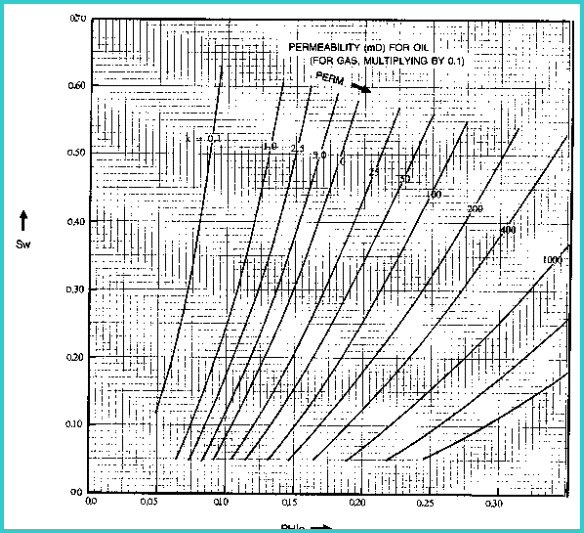Permeability from the Wylie-Rose Method
This is one of the oldest permeability methods available, and is reliable when calibrated to core data.

1: PERMw = CPERM * (PHIe ^ DPERM) / (SWir ^ EPERM)

Where:
CPERM = permeability constant (fractional)
DPERM = porosity exponent (fractional)

EPERM = irreducible saturation exponent (fractional)
PERMw = permeability (millidarcies)
PHIe = effective porosity from any method (fractional)
SWir = irreducible water saturation (fractional)COMMENTS:
Reference:
1. Using Log-Derived Values of Water Saturation and Porosity,
R.L. Morris, W.P. Biggs, SPWLA, 1967.

2. An Investigation of Permeability, Porosity, and Residual Water Saturation Relationships,
A. Timur, SPWLA, 1968

Different researchers have found a variety of exponents based on core analysis studies, and the analyst may find many more in the literature or company files.RECOMMENDED PARAMETERS:
RESEARCHER                   CPERM              DPERM   EPERM
OIL   GAS
Morris-Biggs                   65000 6500              6.0           2.0
Timur                               3400 340                4.4           2.0NUMERICAL EXAMPLE:
Assume data from Classic Example Sand B.
PHIe = 0.30
SWir = Sw = 0.25

Wylie-Rose formula / Morris-Biggs parameters:
CPERM = 62500
DPERM = 6.0
EPERM = 2.0
PERMw = 62500 * (0.30 ^ 6) / (0.25 ^ 2) = 730 mdPermeability from Morris - Biggs parametersPermeability from Timur parametersMETA/LOG "PERM"  Compare Permeability Calculated from Various Methods